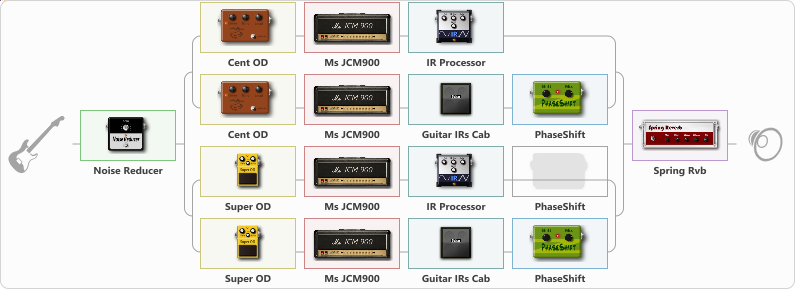# Guit-Rit

Discussion in 'ToneLib-GFX presets' started by carlinos3, Apr 2, 2021.

1. Guit-Rit

Preset name: Djently cruching

Effects chain:Effect: "Noise Reducer" (Dinámica / Filtro), active - "yes"
{
"Sens" = 80
"Mode" = Hard
}

Effect: "Splitter" (Dinámica / Filtro)
{
"A-Bypass" = Off
"A-Pan" = 50
"A-Level" = 70
"B-Bypass" = Off
"B-Pan" = -50
"B-Level" = 70

'A' branch:
{

Effect: "Splitter" (Dinámica / Filtro)
{
"A-Bypass" = Off
"A-Pan" = -39
"A-Level" = 55
"B-Bypass" = Off
"B-Pan" = 2
"B-Level" = 55

'A' branch:
{

Effect: "Cent OD" (Overdrive / Distorsión), active - "yes"
{
"Drive" = 68
"Tone" = 42
"Level" = 59
}

{
"Gain" = 42
"Bass" = 17
"Middle" = 14
"Treble" = 36
"Presence" = 67
"Master" = 70
"Level (dB)" = 2
}

Effect: "IR Processor" (Gabinetes), active - "yes"
{
"IR" = charv.1_dc
"Low Cut (Hz)" = 0
"Hi Cut (kHz)" = 20.0
"Mix" = 100
"Level (dB)" = 5
}
}
'B' branch:
{

Effect: "Cent OD" (Overdrive / Distorsión), active - "yes"
{
"Drive" = 68
"Tone" = 42
"Level" = 59
}

{
"Gain" = 42
"Bass" = 17
"Middle" = 14
"Treble" = 36
"Presence" = 67
"Master" = 70
"Level (dB)" = -2
}

Effect: "Guitar IRs Cab" (Gabinetes), active - "yes"
{
"Model" = Marshall JCM2000 (4x12")
"Mic Position" = Middle
"Mic Distance" = Middle
"Low Cut (Hz)" = 0
"Hi Cut (kHz)" = 20.0
"Mix" = 100
"Level (dB)" = 0
}

Effect: "PhaseShift" (Modulación / Sfx), active - "yes"
{
"Shift" = 36
"Mix" = 100
}
}
}
}
'B' branch:
{

Effect: "Splitter" (Dinámica / Filtro)
{
"A-Bypass" = Off
"A-Pan" = 35
"A-Level" = 55
"B-Bypass" = Off
"B-Pan" = 65
"B-Level" = 55

'A' branch:
{

Effect: "Super OD" (Overdrive / Distorsión), active - "yes"
{
"Drive" = 17
"Tone" = 72
"Level" = 91
}

{
"Gain" = 42
"Bass" = 35
"Middle" = 14
"Treble" = 36
"Presence" = 67
"Master" = 70
"Level (dB)" = 3
}

Effect: "IR Processor" (Gabinetes), active - "yes"
{
"IR" = charv.1_dc
"Low Cut (Hz)" = 0
"Hi Cut (kHz)" = 20.0
"Mix" = 100
"Level (dB)" = 6
}

Effect: "PhaseShift" (Modulación / Sfx), active - "no"
{
"Shift" = 54
"Mix" = 100
}
}
'B' branch:
{

Effect: "Super OD" (Overdrive / Distorsión), active - "yes"
{
"Drive" = 20
"Tone" = 62
"Level" = 91
}

{
"Gain" = 42
"Bass" = 22
"Middle" = 31
"Treble" = 50
"Presence" = 67
"Master" = 70
"Level (dB)" = -2
}

Effect: "Guitar IRs Cab" (Gabinetes), active - "yes"
{
"Model" = Mesa Rectifier (4x12")
"Mic Position" = Middle
"Mic Distance" = Near
"Low Cut (Hz)" = 0
"Hi Cut (kHz)" = 20.0
"Mix" = 100
"Level (dB)" = 0
}

Effect: "PhaseShift" (Modulación / Sfx), active - "yes"
{
"Shift" = 71
"Mix" = 100
}
}
}
}
}

Effect: "Spring Rvb" (Reverberación), active - "yes"
{
"Time" = 7.0
"PreDelay" = 0
"LoDamp" = 16
"HiDamp" = 30
"Mix" = 40
}

Note: You will need to download and install the ToneLib-GFX software to use the preset.

#### Attached Files:

• ###### Guit-Rit.tlgfx
File size:
118.6 KB
Views:
2,380
Sergejs Matvejenkovs likes this.